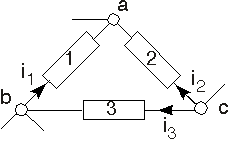# 3.16: Power Conservation in Circuits

$$\newcommand{\vecs}{\overset { \rightharpoonup} {\mathbf{#1}} }$$ $$\newcommand{\vecd}{\overset{-\!-\!\rightharpoonup}{\vphantom{a}\smash {#1}}}$$$$\newcommand{\id}{\mathrm{id}}$$ $$\newcommand{\Span}{\mathrm{span}}$$ $$\newcommand{\kernel}{\mathrm{null}\,}$$ $$\newcommand{\range}{\mathrm{range}\,}$$ $$\newcommand{\RealPart}{\mathrm{Re}}$$ $$\newcommand{\ImaginaryPart}{\mathrm{Im}}$$ $$\newcommand{\Argument}{\mathrm{Arg}}$$ $$\newcommand{\norm}{\| #1 \|}$$ $$\newcommand{\inner}{\langle #1, #2 \rangle}$$ $$\newcommand{\Span}{\mathrm{span}}$$ $$\newcommand{\id}{\mathrm{id}}$$ $$\newcommand{\Span}{\mathrm{span}}$$ $$\newcommand{\kernel}{\mathrm{null}\,}$$ $$\newcommand{\range}{\mathrm{range}\,}$$ $$\newcommand{\RealPart}{\mathrm{Re}}$$ $$\newcommand{\ImaginaryPart}{\mathrm{Im}}$$ $$\newcommand{\Argument}{\mathrm{Arg}}$$ $$\newcommand{\norm}{\| #1 \|}$$ $$\newcommand{\inner}{\langle #1, #2 \rangle}$$ $$\newcommand{\Span}{\mathrm{span}}$$

##### Learning Objectives
• Proof that circuits conserve power regardless of the elements used to construct them.

Now that we have a formal method---the node method---for solving circuits, we can use it to prove a powerful result: KVL and KCL are all that are required to show that all circuits conserve power, regardless of what elements are used to build the circuit.Figure 3.16.1 Part of a general circuit to prove Conservation of Power

First of all, define node voltages for all nodes in a given circuit. Any node chosen as the reference will do. For example, in the portion of a large circuit depicted above, we define node voltages for nodes a, b and c. With these node voltages, we can express the voltage across any element in terms of them. For example, the voltage across element 1 is given by:

$v_{1}=e_{b}-e_{a} \nonumber$

The instantaneous power for element 1 becomes:

$v_{1}i_{1}=(e_{b}-e_{a})i_{1}=e_{b}i_{1}-e_{a}i_{1} \nonumber$

Writing the power for the other elements, we have

$v_{2}i_{2}=e_{c}i_{2}-e_{a}i_{2} \nonumber$

$v_{3}i_{3}=e_{c}i_{3}-e_{b}i_{3} \nonumber$

When we add together the element power terms, we discover that once we collect terms involving a particular node voltage, it is multiplied by the sum of currents leaving the node minus the sum of currents entering. For example, for node b, we have:

$e_{b}(i_{3}-i_{1}) \nonumber$

We see that the currents will obey KCL that multiply each node voltage. Consequently, we conclude that the sum of element powers must equal zero in any circuit regardless of the elements used to construct the circuit.

$\sum_{k}v_{k}i_{k}=0 \nonumber$

The simplicity and generality with which we proved this results generalizes to other situations as well. In particular, note that the complex amplitudes of voltages and currents obey KVL and KCL, respectively. Consequently, we have that:

$\sum_{k}V_{k}I_{k}=0 \nonumber$

Furthermore, the complex-conjugate of currents also satisfies KCL, which means we also have:

$\sum_{k}V_{k}\overline{I_{k}}=0 \nonumber$

And finally, we know that evaluating the real-part of an expression is linear. Finding the real-part of this power conservation gives the result that average power is also conserved in any circuit.

$\sum_{k}\frac{1}{2}\Re (V_{k}\overline{I_{k}})=0 \nonumber$

##### Note

This proof of power conservation can be generalized in another very interesting way. All we need is a set of voltages that obey KVL and a set of currents that obey KCL. Thus, for a given circuit topology (the specific way elements are interconnected), the voltages and currents can be measured at different times and the sum of v-i products is zero.

$\sum_{k}v_{k}(t_{1})i_{k}(t_{2})=0 \nonumber$

Even more interesting is the fact that the elements don't matter. We can take a circuit and measure all the voltages. We can then make element-for-element replacements and, if the topology has not changed, we can measure a set of currents. The sum of the product of element voltages and currents will also be zero!

This page titled 3.16: Power Conservation in Circuits is shared under a CC BY 1.0 license and was authored, remixed, and/or curated by Don H. Johnson via source content that was edited to the style and standards of the LibreTexts platform; a detailed edit history is available upon request.